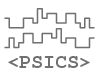PSICS - the Parallel Stochastic Ion Channel Simulator
previous ←  → next
Under development
Please let us know of errors, unclear text or if you have suggestions form improvements:# Passive propagation

Source code of the subroutine that updates the membrane potential. The local inputs are the current membrane potential, any current or voltage clamps on the cell, and the effective conductance and reversal potential that the ion channels provide for each compartment.

The other input quantities define the electrical structure of the discretized cell and are fixed throughout the calculation.

### Fixed quantities

Declarations of structural quantities: these are fixed throughout the calculation

```        integer, intent(in) :: ncompartments, nconnections, ncc, nvc
integer, dimension(nconnections), intent(in) :: con_from, con_to
real, dimension(nconnections), intent(in) :: con_conductance, con_capacitance
real, dimension(ncompartments) :: cpt_capacitance
real, intent(in) :: dt, ftimediff
integer, dimension(ncc), intent(in) :: pos_ccs
integer, dimension(nvc), intent(in) :: pos_vcs

```

### Local inputs

The effective conductance and reversal potential arising from combining all the channels on each compartment, and the values ov current clamps and voltage clamps.

```        real, dimension(ncompartments), intent(in)  :: gchan, echan
real, dimension(ncc), intent(in) :: ccvals
real, dimension(nvc), intent(in) :: vcvals

```

### Work arrays and output

The diag, rhs, and offdiag are internal work arrays. v is the membrane potential: both input and output

```        real, dimension(ncompartments), intent(inout) :: v
real, dimension(ncompartments) :: diag, rhs
real, dimension(nconnections) :: offdiag
real :: fcn, a, b
integer :: i, i_from, i_to, icpt
real :: f

```

### Set up

Populate the matrices and rhs vector. The matrix elements are stored in the diag and offdiag arrays. The off-diagonal elements are stored by connection rather than by compartment.

```        v(pos_vcs) = vcvals;
do i = 1, ncompartments
a = dt * gchan(i)
rhs(i) = a * (echan(i) - v(i))
diag(i) = cpt_capacitance(i) + fcn * a
end do

do i = 1, ncompartments
a = dt * gchan(i)
rhs(i) = a * (echan(i) - v(i))
diag(i) = cpt_capacitance(i) + fcn * a
end do

do i = 1, nconnections
a = dt * con_conductance(i)
offdiag(i) = -fcn * (con_capacitance(i) + a)
end do

rhs(pos_ccs) = rhs(pos_ccs) + dt * ccvals;

do i = 1, nconnections
b = dt * con_conductance(i) * (v(con_from(i)) - v(con_to(i)))
rhs(con_to(i)) = rhs(con_to(i)) + b
rhs(con_from(i)) = rhs(con_from(i)) - b
diag(con_to(i)) = diag(con_to(i)) - offdiag(i)
diag(con_from(i)) = diag(con_from(i)) - offdiag(i)
end do

diag(pos_vcs) = 1.
rhs(pos_vcs) = 0;

```

### Forward sweep

Eliminates points to the right of the leading diagonal, working from the bottom up so that no new elemnts are introduced

```        do i = nconnections, 1, -1
i_from = con_from(i)
i_to = con_to(i)
f = offdiag(i) / diag(i_to)
rhs(i_from) = rhs(i_from) - f * rhs(i_to)
diag(i_from) = diag(i_from) - f * offdiag(i)
end do

diag(pos_vcs) = 1.
rhs(pos_vcs) = 0;

```

### Backsubstitute

The only complication here is that because the loop is over connections, not compartments, it is necessary to check for when a complete row has been finished in order to normalize by the diagonal.

```        icpt = 1
do i = 1, nconnections
if (con_to(i) .ne. icpt) then
rhs(icpt) = rhs(icpt) / diag(icpt)
icpt = con_to(icpt)
end if
rhs(con_to(i)) = rhs(con_to(i)) - offdiag(i) * rhs(con_from(i))
end do
rhs(ncompartments) = rhs(ncompartments) / diag(ncompartments)

v = v + rhs

```

The last step above is to increment v by the deltaV values that are in the rhs array.

previous ←  → next